# Math for the Master(y)

Silver Volume now available in
individual spiral-bound units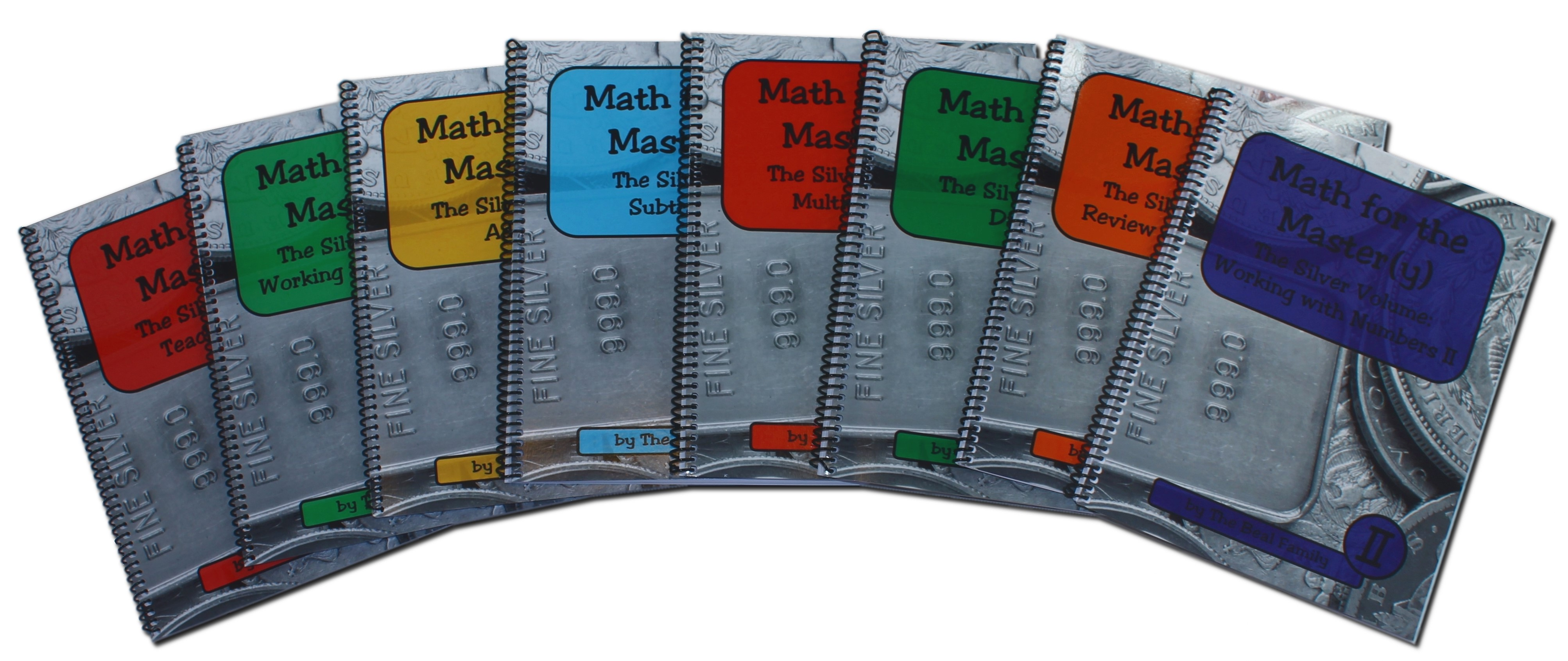Features:

• Able to be used by multiple children in a family
• Short lessons, integrates easily with daily life
• Clear directions and examples written to the child
• No more than one new concept introduced per lesson
• Large print and uncluttered pages
• Family-friendly word problemsSample Pages Teaching Helps includes suggestions for success; FAQ; poster pages on comparing, telling time, measuring, counting money, solving word problems, and more; progress chart and certificates; topical index for all units. Print version is 8.5″ x 11″, spiral bound, 74 pages, \$16.00. PDF version is \$12.00.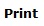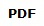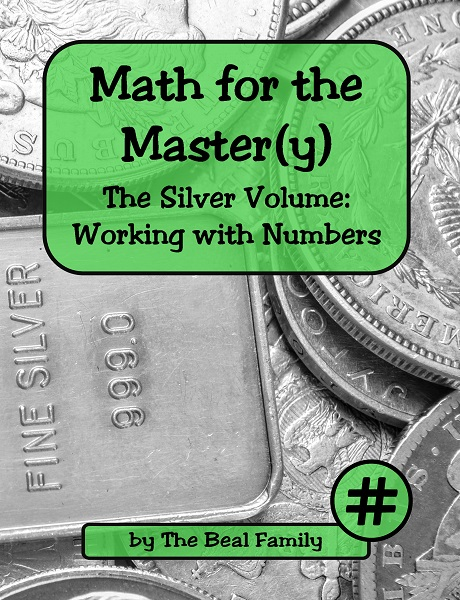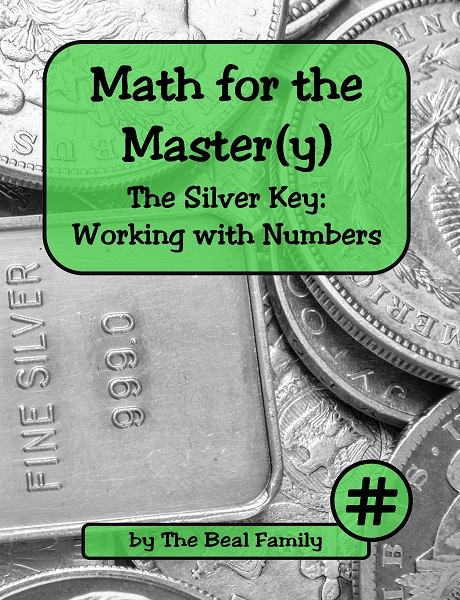Working with Numbers covers counting and writing numbers and their names (0-100); finding smallest and largest; learning less than / greater than; counting by twos, fives, tens, and hundreds; introducing place values; identifying cardinal and ordinal numbers. Print version is 8.5″ x 11″, spiral bound, 78 lessons, 85 pages, \$34.00. PDF version is \$24.00.Addition covers learning and reviewing addition fact families (0-9), solving word problems, adding multi-digit numbers, learning to carry, figuring mentally, checking, and using a calculator to add. Print version is 8.5″ x 11″, spiral bound, 80 lessons, 100 pages, \$36.00. PDF version is \$26.00.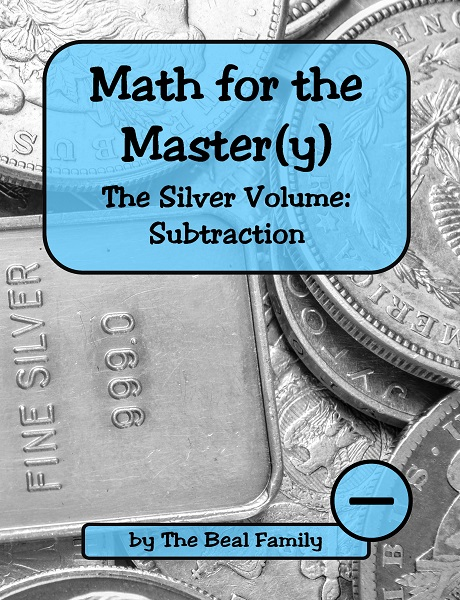Subtraction covers learning and reviewing subtraction fact families (0-9), solving word problems, subtracting multi-digit numbers, learning to borrow, figuring mentally, checking, and using a calculator to subtract. Print version is 8.5″ x 11″, spiral bound, 85 lessons, 109 pages, \$36.00. PDF version is \$26.00.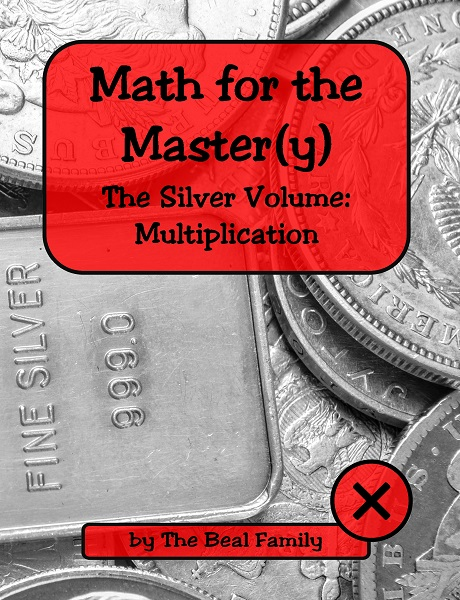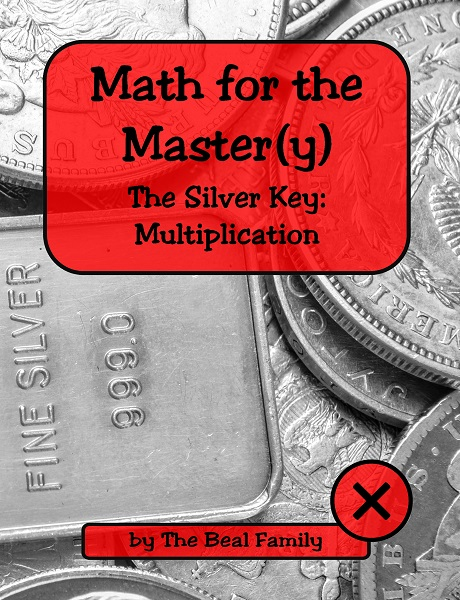Multiplication covers learning and reviewing multiplication fact families (0-12), learning to carry, multiplying larger numbers, solving word problems, multiplying by 10, 100, and 1,000, checking, and using a calculator to multiply. Print version is 8.5″ x 11″, spiral bound, 92 lessons, 120 pages, \$38.00. PDF version is \$28.00.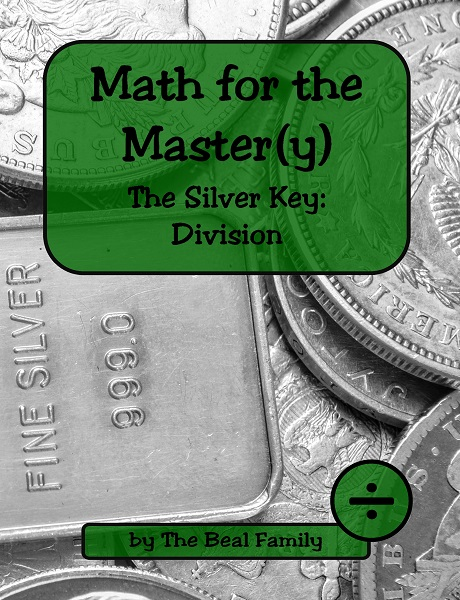Division covers learning and reviewing division fact families (0-12), dividing larger numbers, dividing with a remainder, estimating and rounding to the nearest 10, 100, and 1,000, solving word problems, checking, and using a calculator to divide. Print version is 8.5″ x 11″, spiral bound, 88 lessons, 120 pages, \$38.00. PDF version is \$28.00.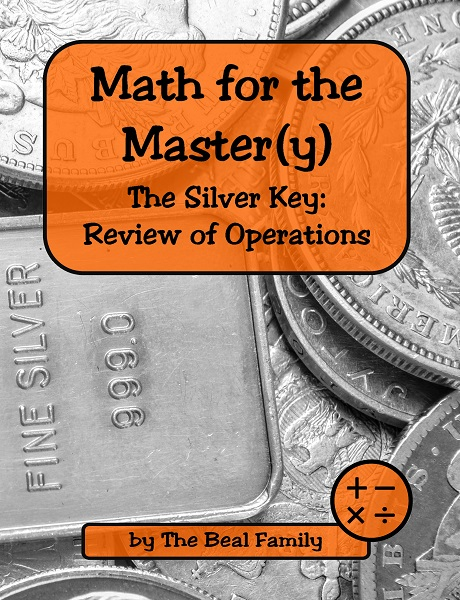Review of Operations covers recalling addition, subtraction, multiplication, and division fact families (timed), answering questions about the processes of addition, subtraction, multiplication, and division, reviewing mixed facts (timed), and solving word problems which are multi-step and/or require a variety of operations. Print version is 8.5″ x 11″, spiral bound, 50 lessons, 77 pages, \$34.00. PDF version is \$24.00.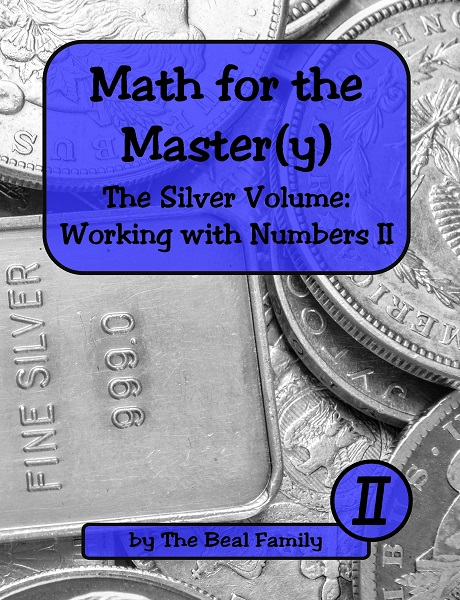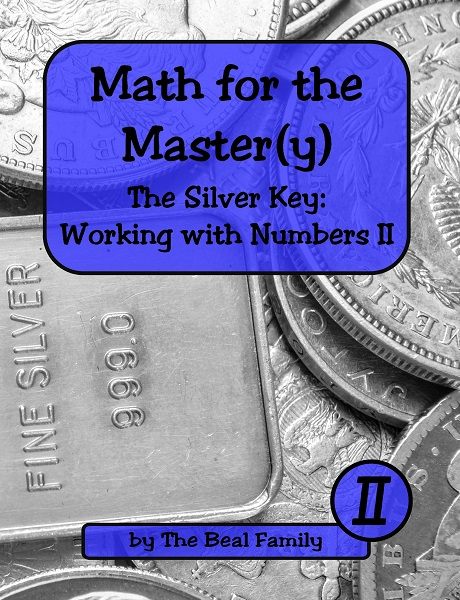Working with Numbers II covers learning about numbers and place values for 1,000 and larger, adding and subtracting large numbers mentally, ordering large numbers, learning Roman numerals, solving problems with mixed operations, learning about mean, median, and mode, figuring with timelines, and drawing and understanding graphs. Print version is 8.5″ x 11″, spiral bound, 86 lessons, 134 pages, \$40.00. PDF version is \$30.00.